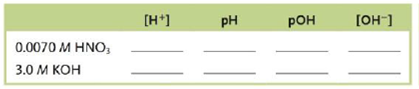# Complete the table for each of the following solutions:### Chemistry: An Atoms First Approach

2nd Edition
Steven S. Zumdahl + 1 other
Publisher: Cengage Learning
ISBN: 9781305079243

#### Solutions

Chapter
Section### Chemistry: An Atoms First Approach

2nd Edition
Steven S. Zumdahl + 1 other
Publisher: Cengage Learning
ISBN: 9781305079243
Chapter 13, Problem 163CWP
Textbook Problem
1 views

## Complete the table for each of the following solutions:(I)

Interpretation Introduction

Interpretation: The missing information in the given table is to be stated.

Concept introduction: The pH is the numeric scale used to measure the [H+] of any solution.

The pOH is the numeric scale used to measure the [OH] of any solution.

The [H+] represents the total hydrogen ion concentration.

The [OH] represents the total hydroxide ion concentration.

To determine: The missing values corresponding to 0.0070MHNO3 in the table.

### Explanation of Solution

Explanation

The value of [H+] is 0.0070M_.

Given

The concentration of HNO3 is 0.0070M

The acid HNO3 is a monoprotic acid and furnishes only one H+ in the aqueous solution; therefore, the concentration of HNO3 corresponds to the concentration of [H+].

Thus, the value of [H+] is 0.0070M_.

Explanation

Given

The value of [H+] is 0.0070M.

The pH value is calculated by the formula.

pH=log[H+]

Substitute value of [H+] in the above equation.

pH=log[0.0070]pH=2.15_

The pOH is 11.85_

(II)

Interpretation Introduction

Interpretation: The missing information in the given table is to be stated.

Concept introduction: The pH is the numeric scale used to measure the [H+] of any solution.

The pOH is the numeric scale used to measure the [OH] of any solution.

The [H+] represents the total hydrogen ion concentration.

The [OH] represents the total hydroxide ion concentration.

To determine: The missing values corresponding to 3.0MKOH in the given table.

### Still sussing out bartleby?

Check out a sample textbook solution.

See a sample solution

#### The Solution to Your Study Problems

Bartleby provides explanations to thousands of textbook problems written by our experts, many with advanced degrees!

Get Started

Find more solutions based on key concepts
Blood LDL values of people eating typical, meat-rich Western diets are generally higher than LDL values of vege...

Nutrition: Concepts and Controversies - Standalone book (MindTap Course List)

What is physically exchanged during crossing over?

Human Heredity: Principles and Issues (MindTap Course List)

What is a chemical reaction?

Biology: The Dynamic Science (MindTap Course List)

What is the meaning of orbital overlap in the formation of a covalent bond?

Introductory Chemistry: An Active Learning Approach

Can mariculture make a significant contribution to marine economics?

Oceanography: An Invitation To Marine Science, Loose-leaf Versin

The Earth reflects approximately 38.0% of the incident sunlight from its clouds and surface. (a) Given that the...

Physics for Scientists and Engineers, Technology Update (No access codes included)